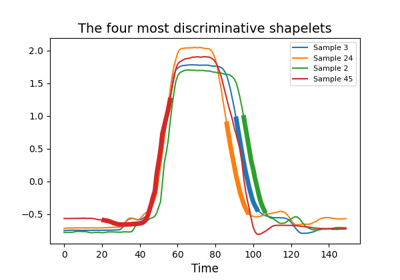# `pyts.transformation`.ShapeletTransform¶

class `pyts.transformation.``ShapeletTransform`(n_shapelets='auto', criterion='mutual_info', window_sizes='auto', window_steps=None, remove_similar=True, sort=False, verbose=0, random_state=None, n_jobs=None)[source]

Shapelet Transform Algorithm.

The Shapelet Transform algorithm extracts the most discriminative shapelets from a data set of time series. A shapelet is defined as a subset of consecutive points from a time series. Two criteria are made available: mutual information and F-scores.

Parameters: n_shapelets : int or ‘auto’ (default = ‘auto’) The number of shapelets to keep. If ‘auto’, n_timestamps // 2 shapelets are considered, where n_timestamps is the number of time points in the dataset. Note that there might be a smaller number of shapelets if fewer than `n_shapelets` shapelets have been extracted during the search. criterion : ‘mutual_info’ or ‘anova’ (default = ‘mutual_info’) Criterion to perform the selection of the shapelets. ‘mutual_info’ uses the mutual information, while ‘anova’ use the ANOVA F-value. window_sizes : array-like or ‘auto ‘(default = ‘auto’) Size of the sliding windows. If ‘auto’, the range for the window sizes is determined automatically. Otherwise, all the elements must be either integers or floats. In the latter case, each element represents the percentage of the size of each time series and must be between 0 and 1; the size of the sliding windows will be computed as `np.ceil(window_sizes * n_timestamps)`. window_steps : None or array-like (default = None) Step of the sliding windows. If None, each `window_step` is equal to 1. Otherwise, all the elements must be either integers or floats. In the latter case, each element represents the percentage of the size of each time series and must be between 0 and 1; the step of the sliding windows will be computed as `np.ceil(window_steps * n_timestamps)`. Must be None if `window_sizes='auto'`. remove_similar : bool (default = True) If True, self-similar shapelets are removed, keeping only the non-self-similar shapelets with the highest scores. Two shapelets are considered to be self-similar if they are taken from the the same time series and have at least one overlapping index. sort : bool (default = False) If True, shapelets are sorted in descending order according to their associated scores. If False, the order is undefined. verbose : int (default = 0) Verbosity level when fitting: if non zero, progress messages are printed. Above 50, the output is sent to stdout. The frequency of the messages increases with the verbosity level. random_state : int, RandomState instance or None (default = None) If int, random_state is the seed used by the random number generator; If RandomState instance, random_state is the random number generator; If None, the random number generator is the RandomState instance used by `np.random`. Only used if `window_sizes='auto'` in order to subsample the dataset to find the best range or if `criterion=='mutual_info'` to add small noise to the data. n_jobs : None or int (default = None) The number of jobs to run in parallel for `fit`. If -1, then the number of jobs is set to the number of cores.

References

  J. Lines, L. M. Davis, J. Hills and A. Bagnall, “A Shapelet Transform for Time Series Classification”. Data Mining and Knowledge Discovery, 289-297 (2012).

Examples

```>>> from pyts.transformation import ShapeletTransform
>>> X = [[0, 2, 3, 4, 3, 2, 1],
...      [0, 1, 3, 4, 3, 4, 5],
...      [2, 1, 0, 2, 1, 5, 4],
...      [1, 2, 2, 1, 0, 3, 5]]
>>> y = [0, 0, 1, 1]
>>> st = ShapeletTransform(n_shapelets=2, window_sizes=)
>>> st.fit(X, y)
ShapeletTransform(...)
>>> len(st.shapelets_)
2
>>> st.indices_.shape
(2, 3)
```
Attributes: shapelets_ : array, shape = (n_shapelets,) The array with the selected shapelets. indices_ : array, shape = (n_shapelets, 3) The indices for the corresponding shapelets in the training set. The first column consists of the indices of the samples. The second column consists of the starting indices (included) of the shapelets. The third column consists of the ending indices (excluded) of the shapelets. scores_ : array, shape = (n_shapelets,) The scores associated to the shapelets. The higher, the more discriminant. If `criterion='mutual_info'`, mutual information scores are reported. If `criterion='anova'`, F-scores are reported. window_range_ : None or tuple Range of the window sizes if `window_sizes='auto'`. None otherwise.

Methods

 `__init__`([n_shapelets, criterion, …]) Initialize self. `fit`(X, y) Fit the model according to the given training data. `fit_transform`(X, y) Fit the model than transform the given training data. `get_params`([deep]) Get parameters for this estimator. `set_params`(**params) Set the parameters of this estimator. `transform`(X) Transform the provided data.
`__init__`(n_shapelets='auto', criterion='mutual_info', window_sizes='auto', window_steps=None, remove_similar=True, sort=False, verbose=0, random_state=None, n_jobs=None)[source]

Initialize self. See help(type(self)) for accurate signature.

`fit`(X, y)[source]

Fit the model according to the given training data.

It finds the `n_shapelets` best shapelets in the training set.

Parameters: X : array-like, shape = (n_samples, n_timestamps) Univariate time series. y : array-like, shape = (n_samples,) Class labels for each data sample. self : object
`fit_transform`(X, y)[source]

Fit the model than transform the given training data.

It finds the `n_shapelets` best shapelets in the training set and computes the distances between them and the training set.

Parameters: X : array-like, shape = (n_samples, n_timestamps) Univariate time series. y : array-like, shape = (n_samples,) Class labels for each data sample. X_new : array, shape = (n_samples, n_shapelets) Distances between the selected shapelets and the samples.
`get_params`(deep=True)

Get parameters for this estimator.

Parameters: deep : bool, default=True If True, will return the parameters for this estimator and contained subobjects that are estimators. params : dict Parameter names mapped to their values.
`set_params`(**params)

Set the parameters of this estimator.

The method works on simple estimators as well as on nested objects (such as `Pipeline`). The latter have parameters of the form `<component>__<parameter>` so that it’s possible to update each component of a nested object.

Parameters: **params : dict Estimator parameters. self : estimator instance Estimator instance.
`transform`(X)[source]

Transform the provided data.

It computes the distances between the selected shapelets and the samples.

Parameters: X : array-like, shape = (n_samples, n_timestamps) Univariate time series. X_new : array, shape = (n_samples, n_shapelets) Distances between the selected shapelets and the samples.

## Examples using `pyts.transformation.ShapeletTransform`¶Shapelet Transform